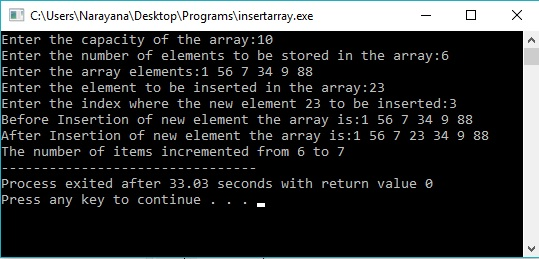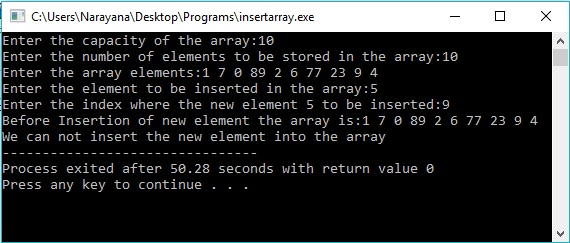# Question & Answer: Write a function named insertInArray that takes the following parameters: list: an integer array arrayCapacity: c…..

Write a function named insertInArray that takes the following parameters: list: an integer array arrayCapacity: capacity of the array numItems: number of items currently in the array index: index at which to insert the new item newVal: value to insert into the array If the array is at capacity then the function should return -1.

Otherwise, insert newVal at index and return 0 for success.

Don't use plagiarized sources. Get Your Custom Essay on
Question & Answer: Write a function named insertInArray that takes the following parameters: list: an integer array arrayCapacity: c…..
GET AN ESSAY WRITTEN FOR YOU FROM AS LOW AS \$13/PAGE

int insertInArray(int list[ ], int arrayCapacity, int* numItems, int index, int newVal)

For example, if the array is {9, 2, 8, 3} and it has the capacity to add a new value of 5 at index 2, then the resulting array should be {9, 2, 5, 8, 3} and the function should return 0. The numItems variable will increment from 4 to 5.

#include<iostream>

using namespace std;

int InsertInarray(int list[],int arrayCapacity,int* numItems,int Index,int newValue) /*function definition InsertInarray()*/

{

int j,i; /* variable declaration*/

if((arrayCapacity-(*numItems))>0) /*if the array capacity is not exceeded then we can insert the element into array*/

{

for(i=Index;i<=(*numItems-1);i++); /* The loop is meant for Identifying the last element’s Index*/

i–; /* getting the desired index where the element to be inserted*/

for(j=i;j>=Index;j–) /* The loop is meant for copying the jth index value into (j+1)th index*/

{

list[j+1]=list[j];

}

list[j+1]=newValue; /*putting the newValue in desired location*/

*numItems+=1; /*Incrementing the number of items in the array*/

return 0; /* returns 0 to the main() function*/

}

else /*if the array capacity is exceeded then we can’t insert the element into array*/

{

return -1; /* returns -1 to the main() function*/

}

}

int main() /*main() function’s definition*/

{

int n,i,k,k1,key,pos,res; /*variable declaration*/

cout<<“Enter the capacity of the array:”; /*prompts the message*/

cin>>n; /*taking the capacity of array a into n*/

int a[n]; /* ‘a’ is the array with maximum of ‘n’ elements */

cout<<“Enter the number of elements to be stored in the array:”; /*prompts the message*/

cin>>k; /* taking the number of elements currently being stored into ‘k’*/

k1=k; /*copies ‘k’ into ‘k1’*/

cout<<“Enter the array elements:”; /*prompts the message*/

for(i=0;i<k;i++) /* The loop is meant for taking the elements into array ‘a’*/

cin>>a[i];

cout<<“Enter the element to be inserted in the array:”; /*prompts the message*/

cin>>key; /* taking the element to be inserted into ‘k’*/

cout<<“Enter the index where the new element “<<key<<” to be inserted:”; /*prompts the message*/

cin>>pos; /* getting the index into ‘pos’, where the ‘key’ is to be inserted*/

cout<<“Before Insertion of new element the array is:”; /*prompts the message*/

for(i=0;i<k;i++) /*displaying the array elements*/

cout<<a[i]<<” “;

res=InsertInarray(a,n,&k,pos,key); /*function call to InsertInarray()*/

if(res==0) /*if res==0*/

{

cout<<“nAfter Insertion of new element the array is:”; /*prompts the message*/

for(i=0;i<k;i++) /*displays the array elements after insertion of a new element*/

cout<<a[i]<<” “;

cout<<“nThe number of items incremented from “<<k1<<” to “<<k; /*displays the element count of the array*/

}

else /* if the size of the array is exeeded */

{

cout<<“nWe can not insert the new element into the array”; /*prompts the message*/

}

return 0;

} /*end of main()*/

Output: